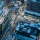Home
IT Knowledge
Inspiration
Languages
EN

# Node.js - global variables

0 points
Created by:p_agon
409

In this article, we would like to show you how to declare and use global variables in Node.js.

Quick solution:

``````global.globalVariable = 'Global variable value.';
console.log(globalVariable); // output: 'Global variable value.'``````

## Introduction

Global variables such as regular variables can be initialized with a value, changed, and even cleared out.

The only difference between a global variable and a regular variable is their scope.

## Practical example

In this example, we create global variable inside example.js file. We can also use and modify it in index.js file.

index.js

``````require('./example.js');

console.log(globalVariable); // output: undefined

globalVariable = 'Value changed in index.js.';
console.log(globalVariable); // output: 'Value changed in index.js.'``````

example.js

``````global.globalVariable = 'Example value initialized in example.js';
console.log(globalVariable); // output: 'Example value initialized in example.js'

globalVariable = 'Value changed in example.js';
console.log(globalVariable); // output: 'Value changed in example.js'

globalVariable = undefined;
console.log(globalVariable); // output: undefined``````

Run the application :

``node index.js``

Output:

``````Example value initialized in example.js
Value changed in example.js
undefined
undefined
Value changed in index.js.``````